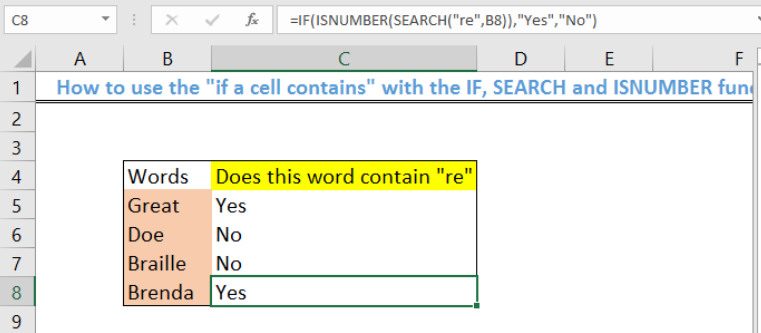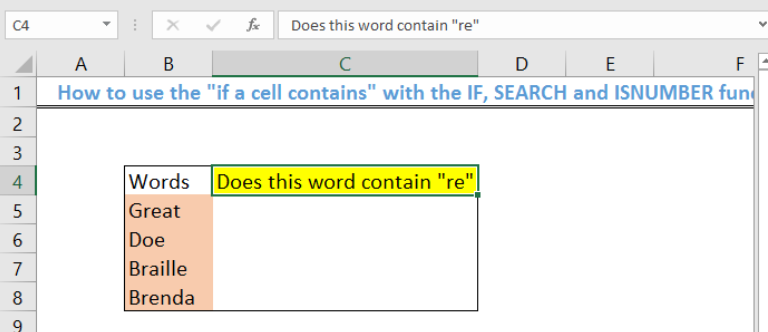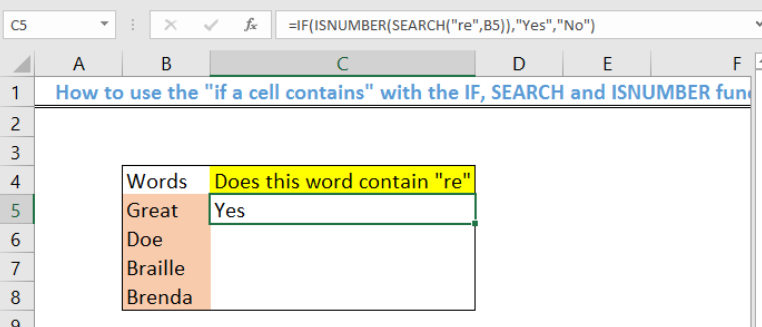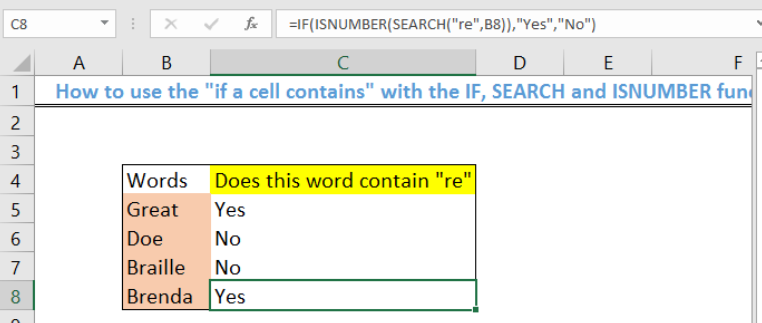Get instant live expert help with Excel or Google Sheets“My Excelchat expert helped me in less than 20 minutes, saving me what would have been 5 hours of work!”

#### Post your problem and you’ll get Expert help in seconds.

Your message must be at least 40 characters
Our professional Expert are available now. Your privacy is guaranteed.

# If cell contains

We can check IF A CELL CONTAINS a specific term in a set of data with a combination of the IF, SEARCH and ISNUMBER functions. We can apply this to copy specific text in another location. The steps below will walk through the process.Figure 1 – Result of using the “if a cell contains” formula

## General Formula

`IF(ISNUMBER(SEARCH"abc",cell_num,[value_if_TRUE],[value_if_FALSE])`

## Formula

`=IF(ISNUMBER(SEARCH("re",B5)),"Yes","No")`

## Setting up the Data

• We will place the list of words in Cell B5 to Cell B8.
• We will name Cell C3 as “Does this word contain “re”. This is what we wish to find from every cellFigure 2- Setting up the Data

## If a cell contains “re” display “Yes” or “No”

• We will click on Cell C4 where we want the search result to be shown.
• We will insert the formula: `=IF(ISNUMBER(SEARCH("re",B5)),"Yes","No")`
• Now, we will press the Enter key.Figure 3 – Cells containing “re” displays Yes or No

• We will use the fill handle to copy the formula to the other cells to get the resultFigure 4 – Final result after using the fill handle tool

## How to display text containing “re”

With the same data, if we want to display the cell text rather than “Yes” or “No”, then we will follow the process below:

• We will click on Cell C5
• We will insert the formula: `=IF(ISNUMBER(SEARCH("re",B5)),B5,"")`
• If the cell contains “re”, then “Great” will be displayed. If it doesn’t contain “re”, then the output cell will remain emptyFigure 5 – Cells containing “re” displays the full term

## Explanation

`=IF(ISNUMBER(SEARCH("re",B5)),"Yes","No")`

• SEARCH Function

The SEARCH function checks Cell B5 if it contains “re.”

• ISNUMBER function

The ISNUMBER function returns as Yes if the text is found and No if the text is not found.

• IF function
`=IF(B5="re", "Yes", "No")`

The IF function returns the result into the output cell based on the returned result of the ISNUMBER function.

## Instant Connection to an Expert through our Excelchat Service

Most of the time, the problem you will need to solve will be more complex than a simple application of a formula or function. If you want to save hours of research and frustration, try our live Excelchat service! Our Excel Experts are available 24/7 to answer any Excel question you may have. We guarantee a connection within 30 seconds and a customized solution within 20 minutes.

### Did this post not answer your question? Get a solution from connecting with the expert.Another blog reader asked this question today on Excelchat:
Solution examplesI have a column where each cell has letters and numbers. The numbers would range from 10 through 18. I would like to find a formula where I can search the cell for any one of the numbers and return a value from a different cell if it finds one. I currently search for a specific number and the formula works but only for that particular number. The formula I currently have is: =IF(ISNUMBER(SEARCH("16",F10)),G10,"") This does work if it finds the value of 16 in the (F10)cell and then places the value from cell "G10" as the result. If it doesn't find the numbers, it will leave the resultant cell blank.
Solved by O. H. in 60 minsI need a formula to count cells with a value containing the word "arc" or the word "robot". The cells are all in the same column, but some of the cells contain both words so I get duplicates in my count.
Solved by M. W. in 39 minshello i need help creating a formula, if a value does not exsits put 0, if a value exist do nothing
Solved by M. F. in 9 minsCompute the weighted average of students score from a spreadsheet using different weight for each category of marks
Solved by G. E. in 20 minshi there, im making a spread sheet to track progress in a game and would like to create a drop log sheet, i was hoping to use the if function to detect weather a item is logged on a sheet then send the sheet number to a list if so
Solved by K. F. in 60 mins## Subscribe to Excelchat.coAnother blog reader asked this question today on Excelchat: